Nothing special here. It’s just a blog post for summarising my algorithm learning course. Probably this was taught in the University but I don’t remember anything, I have no idea about its definition and applications until I take this course.

# Heap-ordered Binary Tree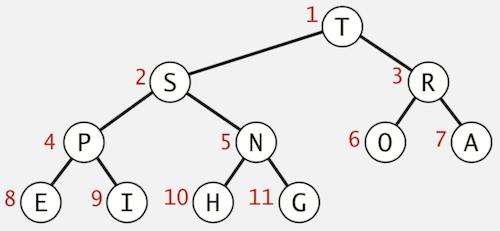• Each node represents a key
• Parent’s key is not smaller than children’s keys

# Array Representation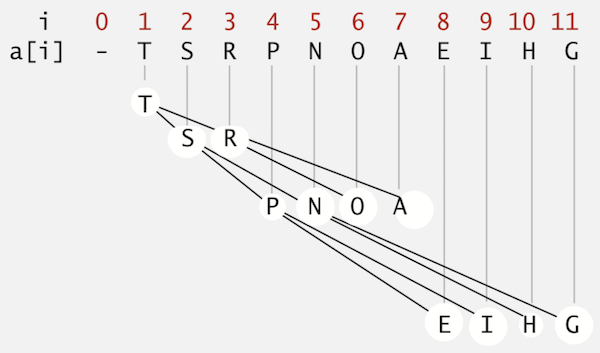• Indices start at 1.
• Take nodes in level order.
• Largest key is a, which is root of binary tree
• Can use array indices to move through tree
• Parent of node at k is at k/2
• Children of node at k are at 2k and 2k+1

# Promotion in a heap

• Scenario: Child’s key becomes larger key than its parent’s key.
• To eliminate the violation:
• Exchange key in child with key in parent.
• Repeat until heap order restored.
• In the below image, the 5th item T is not in the correct order
• T is larger than P (its parent), exchange
• T is still larger than S (its parent), exchange
• Finally, T is in the correct order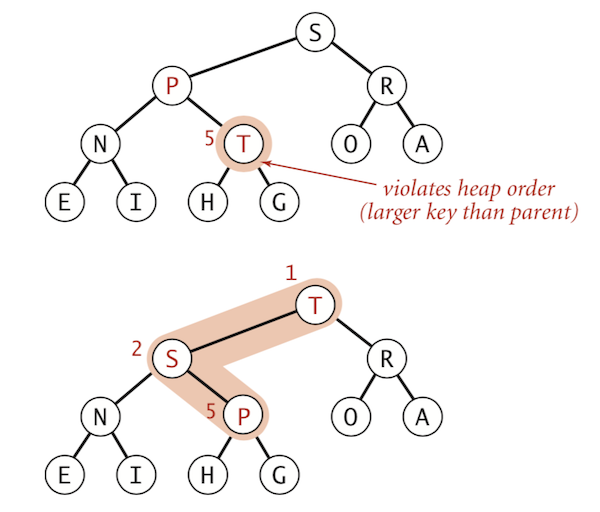private void swim(int k) {
while (k > 1 && less(k/2, k)) {
exch(k, k/2);
k = k/2;
}
}


# Insertion in a heap

• Add node at end, then swim it up.
• Cost: At most 1 + lgN compares.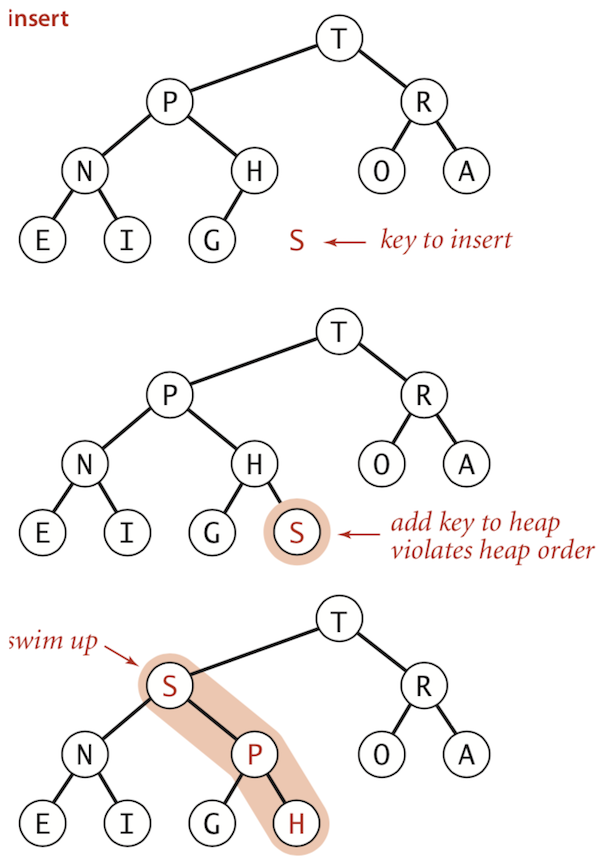public void insert(Key x) {
pq[++N] = x;
swim(N);
}


# Demotion in a heap

• Scenario: Parent’s key becomes smaller than one (or both) of its children’s.
• To eliminate the violation:
• Exchange key in parent with key in larger child.
• Repeat until heap order restored.
• In the below image, the 2nd item H is not in the right order
• H is smaller than its children, exchange with the larger child S
• H is still smaller than its children, exchange with the larger child N
• Finally, H is in the correct orderprivate void sink(int k) {
while (2*k <= N) {
int j = 2*k;
// children of node at k are 2k and 2k+1, decide which one is larger
if (j < N && less(j, j+1)) j++;
// when the item is in the right order, stop
if (!less(k, j)) break;
// otherwise, exchange
exch(k, j);
k = j;
}
}


# Delete the Maximum in a heap

• Exchange root with node at end, then sink it down.
• Cost: At most 2 lgN compares.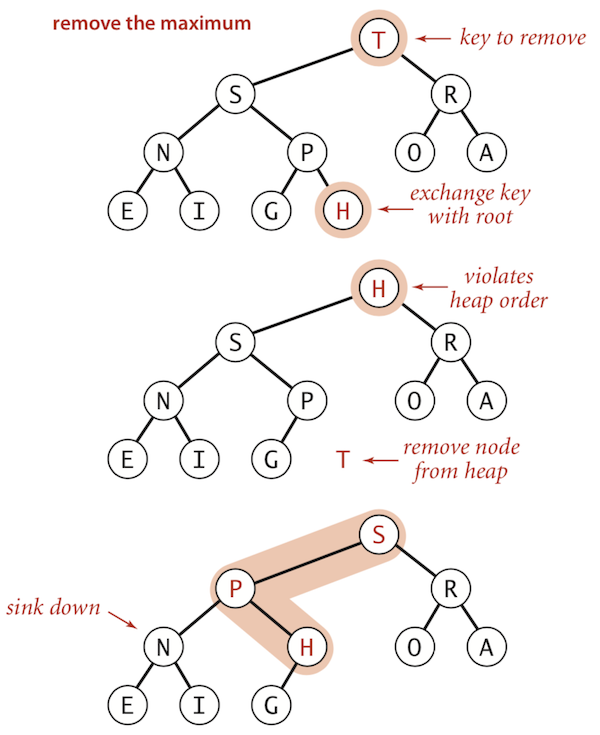public Key delMax() {
Key max = pq;
exch(1, N--);
sink(1);
// prevent lotering
pq[N+1] = null;
return max;
}